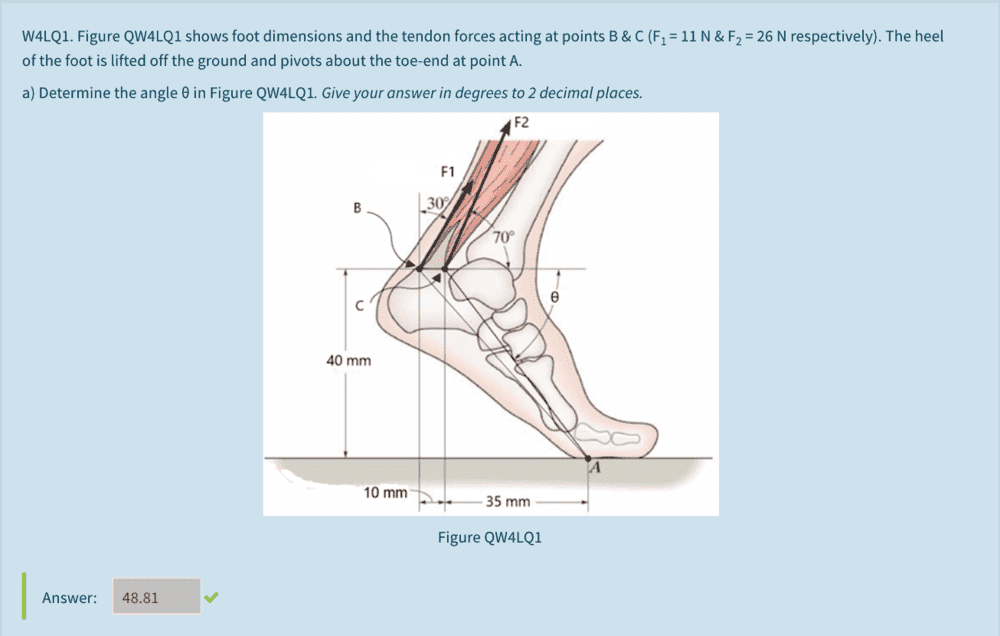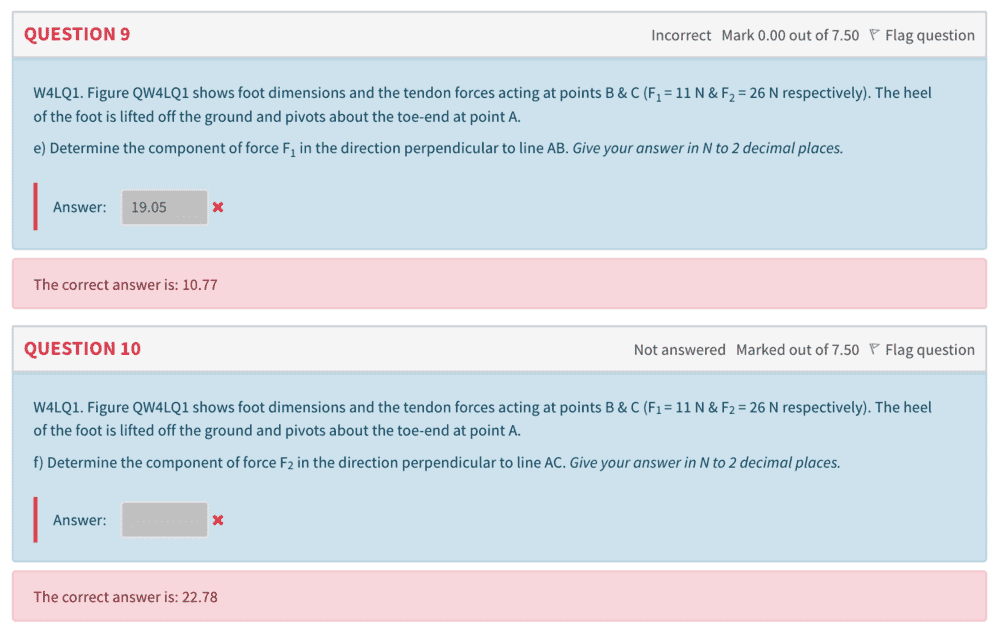# Resolving forces in the direction perpendicular to a line

• Bolter

#### Bolter

Homework Statement
See below
Relevant Equations
N/A
Hi I'm very stuck on what to do for these 2 questions I got wrongCan someone please help me on what triangle I need to sketch out in order to find the 2 components of forces for F1 and F2. I'm assuming you have to make use of the sine or cosine rule here

I'd be grateful for any help given! Thanks

What have you done so far?
Remember that what counts here are the actual forces that are perpendicular to the levers, all respect to the fulcrum A.

•Bolter
What are the horizontal and vertical components of force F1?

I actually managed to find the right answer at the end by drawing triangles and labelling the forces that are perpendicular to the line AB. Then with some basic trig I got the force needed

•Lnewqban